## Dock diving is a great form of athletic competition for dogs of all shapes and sizes. Sheba, the American Pit Bull Terrier, runs and jumps o

Question

Dock diving is a great form of athletic competition for dogs of all shapes and sizes. Sheba, the American Pit Bull Terrier, runs and jumps off the dock with an initial speed of 8.62 m/s at an angle of 28° with respect to the surface of the water. (Assume that the +x axis is in the direction of the run and the +y axis is up.) (a) If Sheba begins at a height of 0.85 m above the surface of the water, through what horizontal distance does she travel before hitting the surface of the water? m

in progress 0
5 months 2021-09-02T12:57:01+00:00 2 Answers 2 views 0

3.139 m

Explanation:

Velocity = 8.62 m/s

Angle of flight = 28°

Height above water = 0.85 m

we are to take the +ve x-axis in the direction of run, and +ve y-axis up.

This is a projectile situation, and the horizontal distance of travel (range) is calculated as

R = (sin2∅)/2g

where g is the acceleration due to gravity = +9.81 m/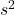sine it acts downwards

R = (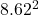sin (2×28°))/(2 x 9.81)

R = (74.30 x 0.8290)/19.62

R = 61.60/19.62 = 3.139 m

62.4m

Explanation:

a) Horizontal distance traveled = x =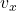* t

where,= horizontal velocity

and t = time in the air)

Time in the air t can be solved using the equation for y: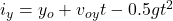where,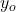= initial height i.e 0.85 m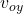= initial vertical velocity

and g = 9.8 m/s^2

When y = 0, the dog has hit the water.

So set y = 0 and solve for t.= 8.62 m/s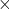(sin 28) = 4.04 m/s

0 = 0.85 m + (4.04 m/s)t – 0.5gt²

0.5t²-4.04t-0.85

Solve this quadratic formula for t: the solutions are t = 8.2 s and t = -0.2. Reject the negative solution, so t = 8.2 s.

How far does the dog travel horizontally in 9.2 s?

x =* t  = (8.62 m/s)(cos 28)8.2 s = 62.4 m